# Dear Family, your Operation Maths guide to Number Sentences and Equations

## Dear Family, your Operation Maths guide to Number Sentences and Equations

Dear Family, below is a brief guide to understanding the topic of number sentences & equations, as well as some practical suggestions as to how you might support your children’s understanding at home. Also below, are a series of links to digital resources that will help both the children, and you, learn more about number sentences & equations.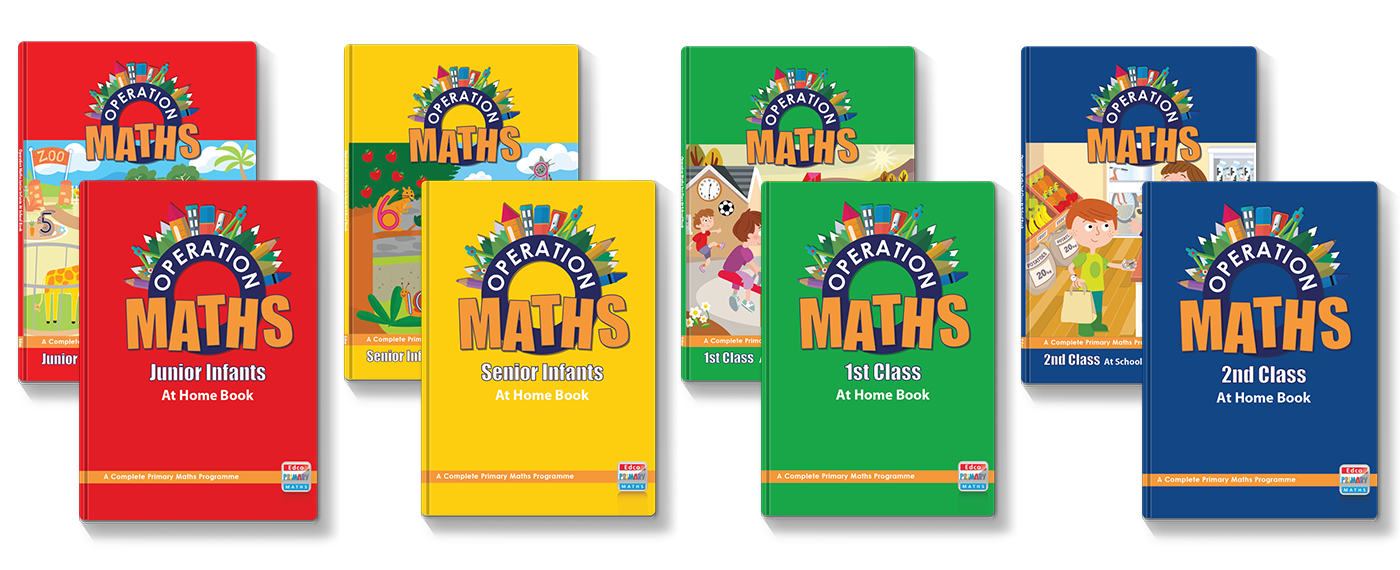You can also find class specific tips in the Operation Maths Dear Family letters for third to sixth class.

#### Understanding Number Sentences and Equations

Number sentences are a part of Algebra. Number sentences are mathematical sentences made up of numbers, symbols and/or signs eg

• 11 – 4 = 7
• 3 × 6 = 18
• 4 + 2 = 5 + 1
• 4 + 5 > 6 + 2
• 5 + ☐ = 9
• 14 – 🌳 = 8
• y + 5 = 20

They can use simple numbers or be more complicated with bigger numbers, letters, fractions etc. Number sentences can be equations, where both sides are equal/balanced (4 + 2 = 5 + 1), or be unequal (4 + 5 > 6 + 2) where one side is greater than or less than the other side. Sometimes, the children are given complete number sentences and are asked to work out if they are true or false. More often, in primary maths, number sentences will have a frame or box to represent the missing value/number which must be worked out or solved e.g. 5 + ☐ = 9. The missing value may also be represented by a symbol or a letter (called a variable) eg 14 – 🌳 = 8, y + 5 = 20

An essential aspect of exploring number sentences is reading and interpreting correctly the signs and symbols. However, for children, the signs and symbols can be very confusing, because they can be so similar and yet all mean something completely different. Take for example + and ×; they are actually the same shape, but turned to look different. And they have very different meanings; the first means add and the second means multiply. Consider some of the other common maths signs: = ÷ – < >. These are all very similar to each other in design and shape, but very different in meaning. No wonder children can get confused! That is why, even though the children will come across number sentences in infants to second class, they will not actually explore this topic in depth, until they are in third class and higher.

Of all the signs used in maths, the equals sign (=) is one that is often misinterpreted. The sign itself is made of two identical lines, to indicate “same-ness”. So this sign is saying that the value of whatever comes before it, is identical to, or the same as, the value that comes after it e.g. 7 = 3 + 4. And sometimes there is more than one value on either side of the equals sign, so that each side has to be calculated to see if it is true e.g. 10 – 4 = 3 + 2 + 1 (Tip: the children could write the value for each side above/below that side so that it’s very obvious whether it’s true or not). The horizontal identical lines in the equals sign, also remind us of a balanced scales (see image below), where the total value/weight on both sides, is equal. When reading this sign with your child, always say “equals” or “is the same as”.Number sentences are simply a mathematical way to represent a scenario or story. If I buy three big bars of chocolate, costing €1.50 each, I can show that information with these number sentences:

€1.50 + €1.50 + €1.50

or

3 x €1.50

So, often there can be more than one way to represent the information.

The child may be asked to represent a story or word problem with a number sentence. Or they may be asked to create a suitable story or word problem to match a given number sentence.Whenever your child is working with word problems at home, encourage them to show it as a number sentence first, before they start to solve it. You can also ask them to show you how they sometimes use model drawings or number bond drawings (see image above) at school to help picture the problem. Drawings and diagrams are particularly useful as they encourage the child to see the “big picture” of the maths story, which can be a great help if they are finding the numbers and symbols difficult to interpret.

Sometimes your child might be given number sentences and asked to identify if they are true or false. To do this, it is not always necessary to work out both sides of the number sentence exactly. There is (usually) only one true or correct option, meaning that every other answer is incorrect or false. Encourage the children to use their estimation and number sense skills to quickly recognise when a statement is obviously false, e.g. in (c) below, two hundred and something and one hundred and something is definitely not bigger than 500 so I don’t need to work it out exactly to know that it is false. And while you might think this is a type of ‘cheat’ strategy, in reality, it is about using a more efficient approach, while also emphasising the value of estimation.

Now that you appreciate how confusing maths signs can be, don’t take it for granted that your child does, or will, understand the meaning of all the maths signs that they come across in their books. If your child is working with number sentences, ask them to read the sentence out loud for you and/or to tell you how their teacher verbalises certain signs. In school, we will often use a variety of language to describe a sign and sometimes that depends on the story that goes with the number sentence. For example, with the number sentence 7 x 9, that could be verbalised as seven multiplied by nine, seven groups of nine, seven nines etc. 20 ÷ 2 could be verbalised as twenty divided into two equal groups/parts, twenty divided by two, how many groups of two in twenty, etc.

#### Practical Suggestions for all Children

• Encourage your child to read aloud every number sentence. For example for 11 – 4 = 7 say “eleven subtract/minus/takeaway four equals seven”. Ask them to suggest a matching scenario/story eg “I had 11 sweets and I ate 4, now I have 7 left” or “I have 11 sweets and you have 4, so I have 7 more than you” (ie the difference between our amounts). Always read = as “is the same as” or “equals”.
• Ask your child to suggest how to represent the various maths scenarios that you encounter, as number sentences, e.g. how to work out the cost of a number of some items, the change that would be due after spending a certain amount, the number of sweets that everyone can get when a large bag is shared out, etc. Even if the child does not calculate an answer, it will benefit them to consider how the scenario might be shown using numbers and signs.
• In Operation Maths, the children may be asked to identify the maximum and minimum amounts that could be used in a number sentence. Draw your child’s attention to incidences where maximum and minimum are used in everyday life:
• you have to be a minimum of 1.1m to go on a certain theme park ride
• the maximum number of passengers on the bus is 52
• the maximum speed on a motorway is 120 km/h
• a child must be a minimum of 15 kg to move from an infant seat to a booster seat in the car.
• Whenever your child is working with word (maths story) problems at home, encourage them to show it as a number sentence or to make a drawing/diagram first, before they start to solve it.

#### Digital Resources for Third to Sixth Classes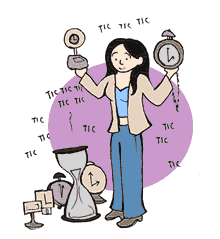Introduction to Algebra: information on basic algebra and how to balance equations from Maths is Fun.Happy Numbers Fifth Grade: Do the activities in Module 5, Algebraic ExpressionsThat Quiz – Arithmetic: Use this to practice different types of number sentences; then try Inequalities (greater than, less than). You can also choose different options from the menu on the left-hand side.I Know it! – Basic Algebra (Fourth Grade): Go to the Basic Algebra section to do any of the activities. You could also try the Basic Algebra section in Fifth Grade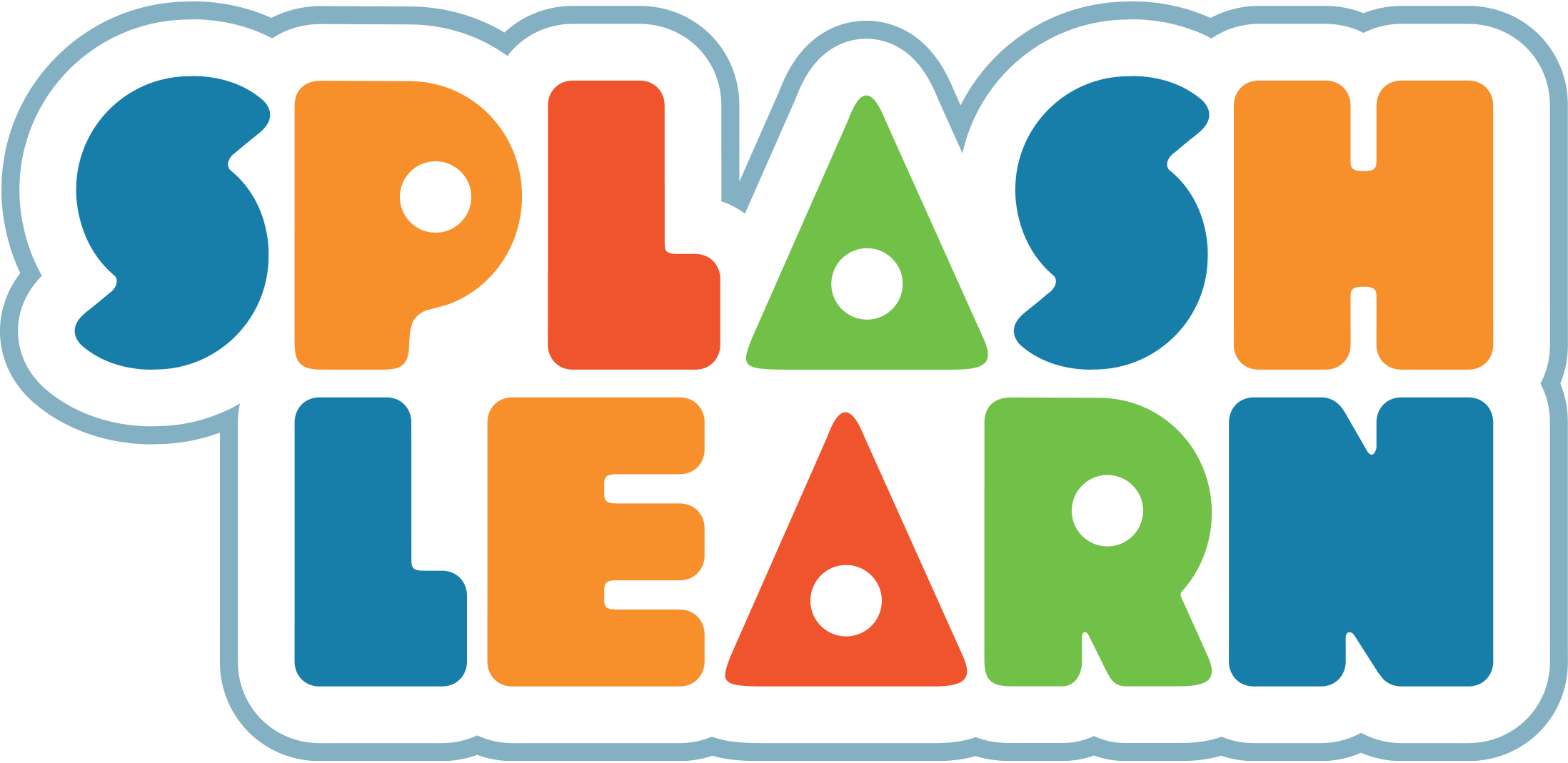Number Sentences: Background information including definitions and examples from Splash LearnWrite expressions: A practice game from Splash Learn. Chose the correct number sentence to match the word sentence.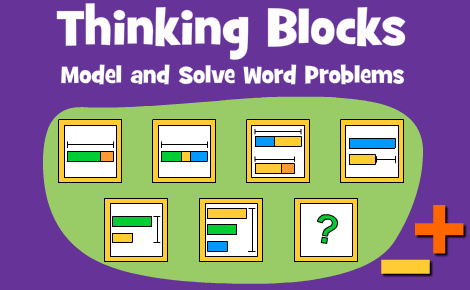Thinking Blocks: Practice making bar models that match word problems and then calculate and answer. Work through these in order, ie start with Thinking Blocks junior and if you feel that this is too easy then move onto the next set.Number Balance: solve the equations by hanging tags on the correct number on the number balance. Has lots of different levels.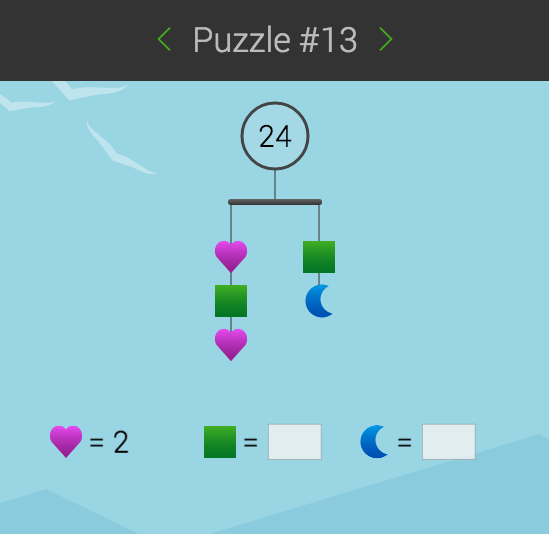SolveMe Mobiles: These are a series of hanging mobiles puzzles that may or may not be balanced, and using the information you have to work out the missing values. They start quite easy and then progress in difficulty. You can register for free so that you can save your progress. Scroll down to the end of the page to play.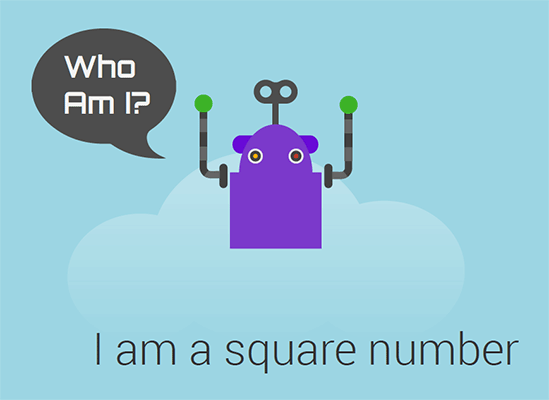SolveMe Who am I: Another series of puzzles; this time you need to work out he mystery number from the clues given. They start quite easy and then progress in difficulty. You can register for free so that you can save your progress.Mashup Math Equation Puzzles: There are lots of colourful puzzles to solve here, often with a seasonal theme. Print them out and solve them or just view them on a screen and see if you can work out the value of the symbols on each line. You can also check out the Mashup Math website for more puzzles, videos etc.Functions and Equations: a selection of practice games from ixl.com. You can do a number of free quizzes each day without having a subscription. Start with the second class games and work up through the activities (Please note that the class levels used here don’t exactly match the class level content in the Irish maths curriculum).